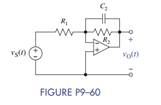Create an Account

Home / Questions / For the inverting OP AMP circuit shown in Figure P9–60, write a differential equation for v O (t)..

For the inverting OP AMP circuit shown in Figure P9–60, write a differential equation for v O (t)..

For the inverting OP AMP circuit shown in Figure P9–60, write a differential equation for vO(t) in terms of the elements and vS(t). Assume vC (0) = 0. Then let vS(t) = e−10t u (t) V, R1 =1kΩ, R2 = 10 kΩ, and C = 1 μF and using Laplace techniques, find the output vO(t).Jul 27 2020 View more View LessSubscribe To Get Solution